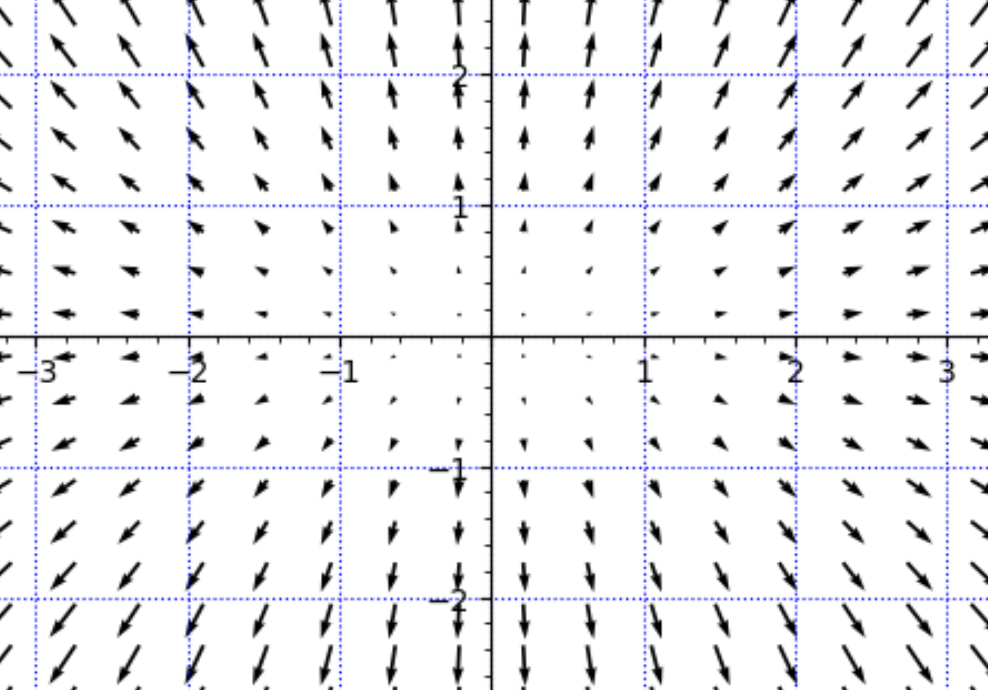# plot_vector_field : unitshi i try this code :

y=var('y')#x l'est déjà par défaut
coef=10
g = plot_vector_field((x*coef, 2*y*coef), (x,-4,4), (y,-3,3),
gridlines=True, gridlinesstyle=dict(color="blue", linestyle=":"),
frame=False, axes=True)
show(g)


As my student discovery vector fields, first we have drawn, by hand on the paper, this vector field :

they have understood that from the point (1,1) they have to draw the vector (1,2), they have counted on the grid to do this precisely

but now on the result :you can see that Sagemath have converted the units in some way, maybe for lisibility, so that the vecteur on (1,1) is only colinear to (1,2) but IS not (1,2).

how can i understand where to customize the factor of conversion that Sagemath uses. Or, at least, to understand how it is calculated ?

edit retag close merge delete